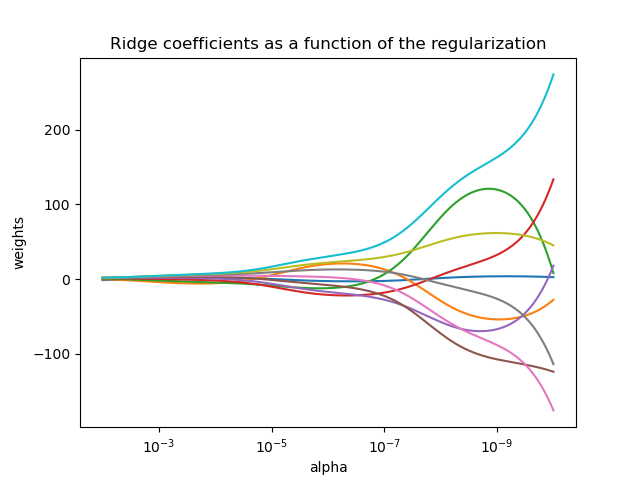# Plot Ridge coefficients as a function of the regularization¶

Shows the effect of collinearity in the coefficients of an estimator.

Ridge Regression is the estimator used in this example. Each color represents a different feature of the coefficient vector, and this is displayed as a function of the regularization parameter.

This example also shows the usefulness of applying Ridge regression to highly ill-conditioned matrices. For such matrices, a slight change in the target variable can cause huge variances in the calculated weights. In such cases, it is useful to set a certain regularization (alpha) to reduce this variation (noise).

When alpha is very large, the regularization effect dominates the squared loss function and the coefficients tend to zero. At the end of the path, as alpha tends toward zero and the solution tends towards the ordinary least squares, coefficients exhibit big oscillations. In practise it is necessary to tune alpha in such a way that a balance is maintained between both.# Author: Fabian Pedregosa -- <fabian.pedregosa@inria.fr>

print(__doc__)

import numpy as np
import matplotlib.pyplot as plt
from sklearn import linear_model

# X is the 10x10 Hilbert matrix
X = 1. / (np.arange(1, 11) + np.arange(0, 10)[:, np.newaxis])
y = np.ones(10)

# #############################################################################
# Compute paths

n_alphas = 200
alphas = np.logspace(-10, -2, n_alphas)

coefs = []
for a in alphas:
ridge = linear_model.Ridge(alpha=a, fit_intercept=False)
ridge.fit(X, y)
coefs.append(ridge.coef_)

# #############################################################################
# Display results

ax = plt.gca()

ax.plot(alphas, coefs)
ax.set_xscale('log')
ax.set_xlim(ax.get_xlim()[::-1])  # reverse axis
plt.xlabel('alpha')
plt.ylabel('weights')
plt.title('Ridge coefficients as a function of the regularization')
plt.axis('tight')
plt.show()


Total running time of the script: ( 0 minutes 0.212 seconds)

Gallery generated by Sphinx-Gallery